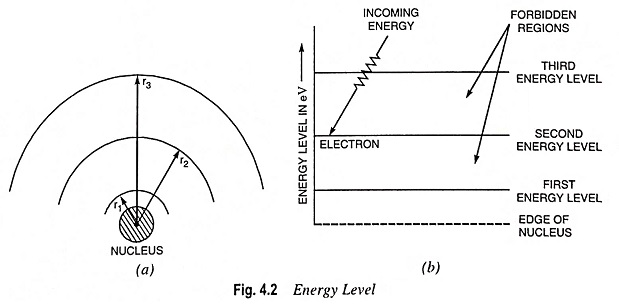## Electron Orbits and Energy Levels:

As already discussed above electron orbits can occupy only certain rings or shells at fixed distances from the nucleus. These shells may be circular or elliptical and are numbered as K, L, M, N, O, P, and Q, K being nearest to the nucleus. Each shell can contain only a particular number of electrons. The formula 2n2 (n being the number of shell or level, the first shell or level being K) determines the number of electrons that can be accommodated in any level. For example Kth level can have maximum of 2 electrons. Similarly L = 2 x 22 = 8, M = 2 x 32 = 18, N = 2 x 42 = 32.

The limitation to the formula is that the number of electrons in the outermost shell of an atom cannot exceed 8. The electrons in the outer shell determine the electrical and chemical characteristics of each particular type of atom and are referred to as valence electrons. An atom may have its outer or valence shell completely filled or only partially filled.

From discussions made above it is clear that electrons can revolve only in the permitted orbits (i.e., orbits of radii r1, r2 and r3) and not in any intermediate orbit. Thus all the radii between r1 and r2 and between r2 and r3 are forbidden (for explanation refer to quantum physics). Each electron orbits has fixed amount of energy associated with it and the electrons moving in a particular orbit possess the energy of that orbit. Thus total energy of an electron can be identified with the size of its orbit—the larger the orbit, the greater is its energy i.e., outer orbit electrons possess more energy than the inner orbit electrons.The level of energy obtainable with different electron orbits can be conveniently determined from Eq. (4.6) and represented by horizontal lines, as illustrated in Fig. 4.2 (b). This is known as en­ergy level diagram. Electrons in the smallest orbit are on the first energy level; electrons in the second orbit are on the second en­ergy level and so on. Thus energy level is just another way of representing the orbital radius.Thus electrons closest to the nucleus need the greatest energy application to extract them from the atom. The electrons in inner orbits, thus, remain bound to the nucleus and are, therefore, called bound electrons.

The energy levels considered above are measured in electron volts (eV).

Between each energy level a forbidden region exists. Electrons cannot orbit in forbidden regions. However, they may quickly pass them. If electrons in a particular orbit acquire enough energy from some outside source (such as an external electric field or thermal energy), they will jump to a high energy level by passing quickly through the forbidden region. A definite amount of energy is required to be spent in order to remove an electron from its orbit (level).

Excited electrons arc unstable, and they tend to fall hack to low energy level by giving up their additional energy. The electrons that do leave their parent atom are termed free electrons.

Scroll to Top